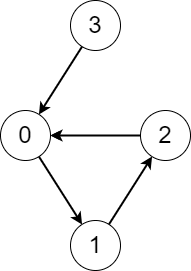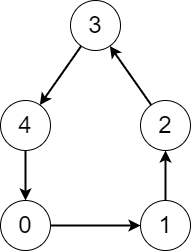# 2876. Count Visited Nodes in a Directed Graph

## Description

There is a directed graph consisting of n nodes numbered from 0 to n - 1 and n directed edges.

You are given a 0-indexed array edges where edges[i] indicates that there is an edge from node i to node edges[i].

Consider the following process on the graph:

• You start from a node x and keep visiting other nodes through edges until you reach a node that you have already visited before on this same process.

Return an array answer where answer[i] is the number of different nodes that you will visit if you perform the process starting from node i.

Example 1:Input: edges = [1,2,0,0]
Output: [3,3,3,4]
Explanation: We perform the process starting from each node in the following way:
- Starting from node 0, we visit the nodes 0 -> 1 -> 2 -> 0. The number of different nodes we visit is 3.
- Starting from node 1, we visit the nodes 1 -> 2 -> 0 -> 1. The number of different nodes we visit is 3.
- Starting from node 2, we visit the nodes 2 -> 0 -> 1 -> 2. The number of different nodes we visit is 3.
- Starting from node 3, we visit the nodes 3 -> 0 -> 1 -> 2 -> 0. The number of different nodes we visit is 4.


Example 2:Input: edges = [1,2,3,4,0]
Output: [5,5,5,5,5]
Explanation: Starting from any node we can visit every node in the graph in the process.


Constraints:

• n == edges.length
• 2 <= n <= 105
• 0 <= edges[i] <= n - 1
• edges[i] != i

## Solutions

• class Solution {
public int[] countVisitedNodes(List<Integer> edges) {
int n = edges.size();
int[] ans = new int[n];
int[] vis = new int[n];
for (int i = 0; i < n; ++i) {
if (ans[i] == 0) {
int cnt = 0, j = i;
while (vis[j] == 0) {
vis[j] = ++cnt;
j = edges.get(j);
}
int cycle = 0, total = cnt + ans[j];
if (ans[j] == 0) {
cycle = cnt - vis[j] + 1;
}
j = i;
while (ans[j] == 0) {
ans[j] = Math.max(total--, cycle);
j = edges.get(j);
}
}
}
return ans;
}
}

• class Solution {
public:
vector<int> countVisitedNodes(vector<int>& edges) {
int n = edges.size();
vector<int> ans(n), vis(n);
for (int i = 0; i < n; ++i) {
if (!ans[i]) {
int cnt = 0, j = i;
while (vis[j] == 0) {
vis[j] = ++cnt;
j = edges[j];
}
int cycle = 0, total = cnt + ans[j];
if (ans[j] == 0) {
cycle = cnt - vis[j] + 1;
}
j = i;
while (ans[j] == 0) {
ans[j] = max(total--, cycle);
j = edges[j];
}
}
}
return ans;
}
};

• class Solution:
def countVisitedNodes(self, edges: List[int]) -> List[int]:
n = len(edges)
ans =  * n
vis =  * n
for i in range(n):
if not ans[i]:
cnt, j = 0, i
while not vis[j]:
cnt += 1
vis[j] = cnt
j = edges[j]
cycle, total = 0, cnt + ans[j]
if not ans[j]:
cycle = cnt - vis[j] + 1
total = cnt
j = i
while not ans[j]:
ans[j] = max(total, cycle)
total -= 1
j = edges[j]
return ans


• func countVisitedNodes(edges []int) []int {
n := len(edges)
ans := make([]int, n)
vis := make([]int, n)
for i := range ans {
if ans[i] == 0 {
cnt, j := 0, i
for vis[j] == 0 {
cnt++
vis[j] = cnt
j = edges[j]
}
cycle, total := 0, cnt+ans[j]
if ans[j] == 0 {
cycle = cnt - vis[j] + 1
}
j = i
for ans[j] == 0 {
ans[j] = max(total, cycle)
total--
j = edges[j]
}
}
}
return ans
}

func max(a, b int) int {
if a > b {
return a
}
return b
}

• function countVisitedNodes(edges: number[]): number[] {
const n = edges.length;
const ans: number[] = Array(n).fill(0);
const vis: number[] = Array(n).fill(0);
for (let i = 0; i < n; ++i) {
if (ans[i] === 0) {
let [cnt, j] = [0, i];
while (vis[j] === 0) {
vis[j] = ++cnt;
j = edges[j];
}
let [cycle, total] = [0, cnt + ans[j]];
if (ans[j] === 0) {
cycle = cnt - vis[j] + 1;
}
j = i;
while (ans[j] === 0) {
ans[j] = Math.max(total--, cycle);
j = edges[j];
}
}
}
return ans;
}